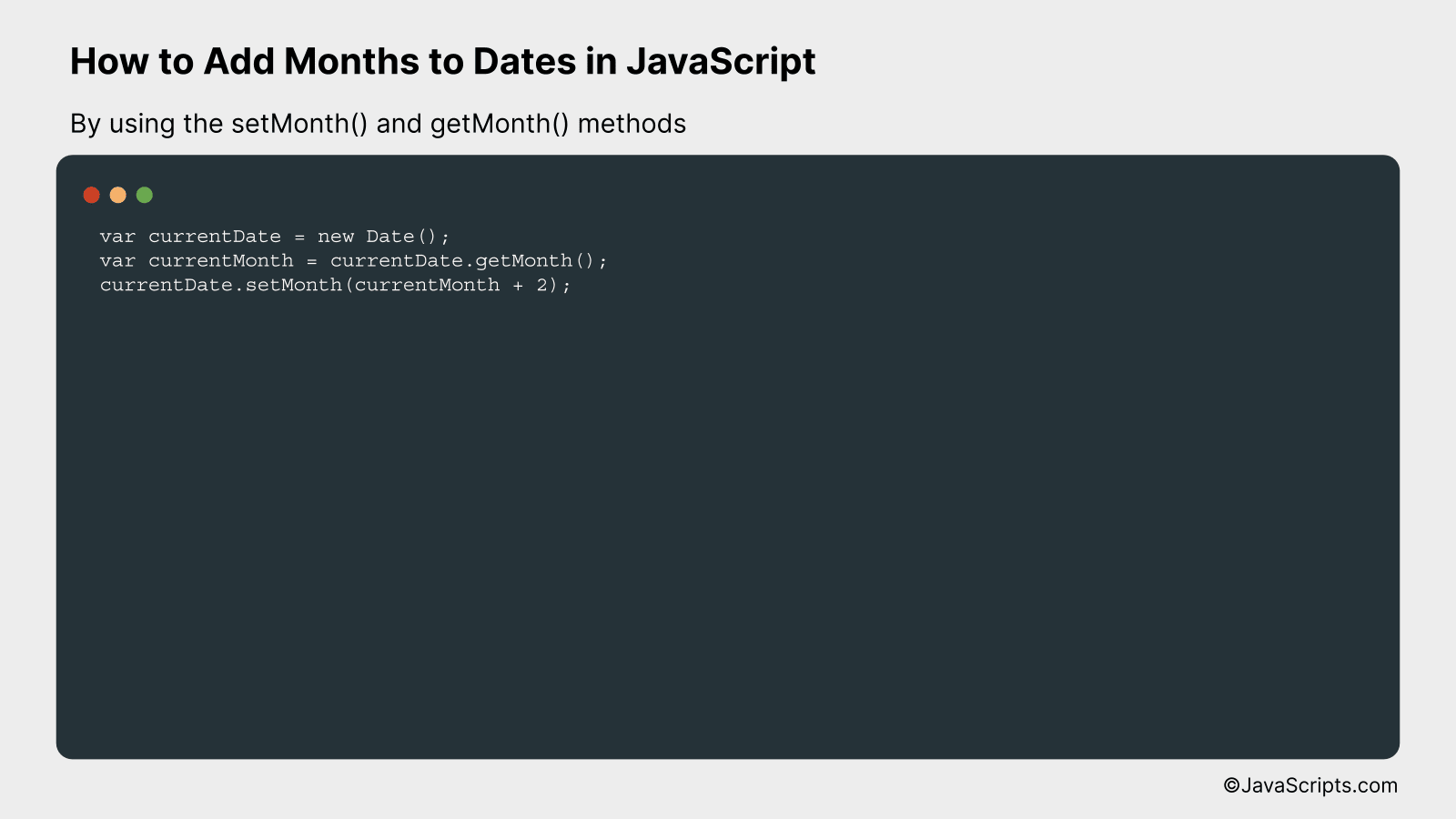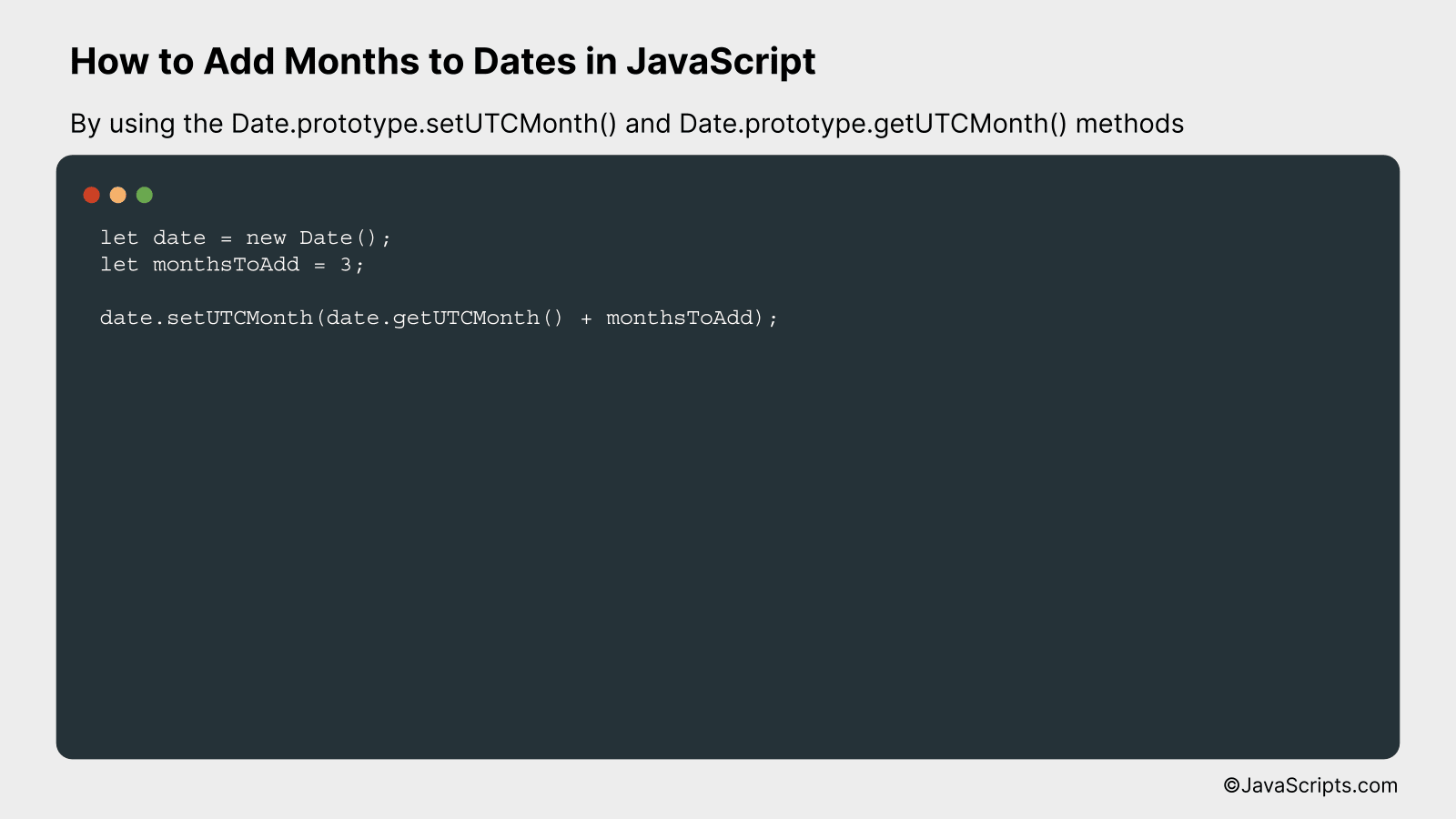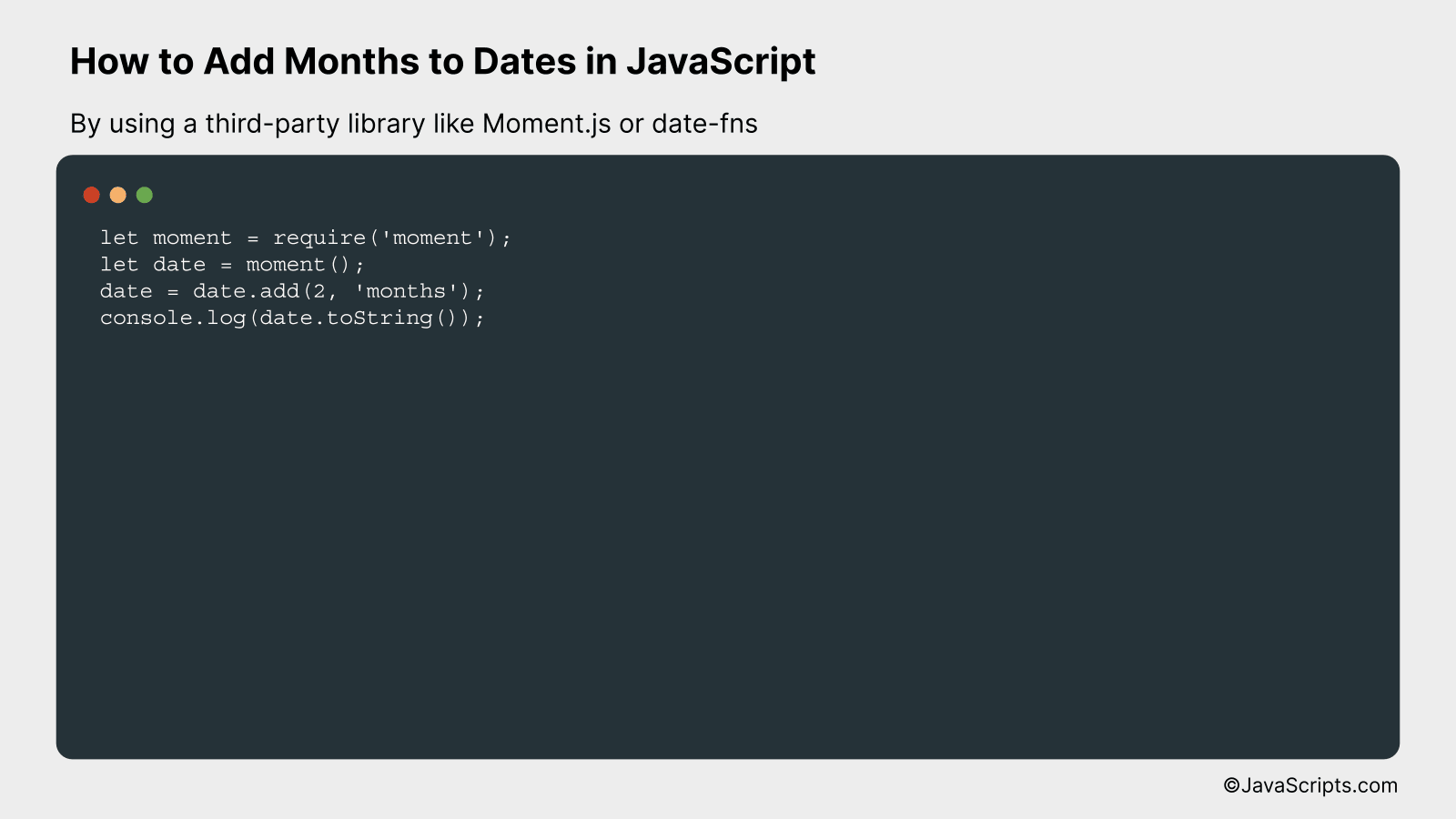# How to Add Months to Dates in JavaScript

If you’ve ever found yourself tangled in a web of date manipulations in JavaScript, you’re not alone. Often, a task as seemingly simple as adding months to a given date can turn into a daunting challenge. But worry not, we’re going to untangle this together!

As we dive into the world of JavaScript dates, you’ll discover how to effortlessly add months to any given date. With step-by-step guidance, we’ll demystify the process, making it easy for you to incorporate into your own coding projects. Don’t worry, this won’t be a complex journey, as we’ll keep things simple and understandable.

In this post, we will be looking at the following 3 ways to add months to dates in JavaScript:

• By using the setMonth() and getMonth() methods
• By using the Date.prototype.setUTCMonth() and Date.prototype.getUTCMonth() methods
• By using a third-party library like Moment.js or date-fns

Let’s explore each…

## #1 – By using the setMonth() and getMonth() methodsWe are going to add months to a given date in JavaScript by using the getMonth() method to find the current month, adding the desired months to it, and then using the setMonth() method to update the original date. We’ll illustrate this with a practical example.

``````
var currentDate = new Date();
var currentMonth = currentDate.getMonth();
currentDate.setMonth(currentMonth + 2);
``````

### How it works

This JavaScript code snippet uses the Date object to get the current date, then retrieves the current month using getMonth(), adds the required months, and updates the date using setMonth().

• Step 1: We create a Date object which holds our current date. The Date object is part of the JavaScript core and provides numerous methods and properties to work with dates.
• Step 2: Using the getMonth() method of the Date object, we get the current month. Note that JavaScript counts months from 0 to 11, where 0 is January and 11 is December.
• Step 3: After retrieving the current month, we add the desired number of months to it. In this example, we have added 2 months.
• Step 4: The updated month value is then passed to the setMonth() method of the Date object which modifies the month of our date accordingly.
• Step 5: The modified date is now stored in the original Date object. We can use this updated date as needed.

## #2 – By using the Date.prototype.setUTCMonth() and Date.prototype.getUTCMonth() methodsThe provided JavaScript code will add a specified number of months to a given date by using the getUTCMonth() method to retrieve the current month of the date, and then the setUTCMonth() method to set a new month that is the sum of the current month and the number of months to add. The demonstration will be conducted using a hypothetical date and month increment.

``````
let date = new Date();

```
```

### How it works

The JavaScript code sample above modifies the specified date by adding a certain number of months. It initially retrieves the current month of the date using the getUTCMonth() method, which returns the month of a date as a zero-based value (where 0 indicates January). It then adds the desired number of months to this value, and applies the result as the new month of the date using the setUTCMonth() method.

• Step 1: The current date is stored in the ‘date’ variable and the number of months to add is stored in ‘monthsToAdd’.
• Step 2: The getUTCMonth() method is called on the ‘date’ object to retrieve the current month. It’s important to note that JavaScript counts months from 0 (January) to 11 (December).
• Step 3: The setUTCMonth() method is then called on the ‘date’ object, passing in the sum of the current month and ‘monthsToAdd’ as an argument. This method sets the month for the ‘date’ object, based on universal time.
• Step 4: If the resulting month exceeds 11 (December), this method will adjust the year accordingly. For example, if the current date is in October (month 9) and ‘monthsToAdd’ is 3, the new date will be in January of the next year.

## #3 – By using a third-party library like Moment.js or date-fnsThis task involves adding a specified number of months to a given date using JavaScript, with the help of libraries like Moment.js or date-fns. An example will clarify the usage of these libraries for date manipulation.

### Moment.js Example

``````
let moment = require('moment');
let date = moment();
console.log(date.toString());
``````

### How it works

This code snippet adds two months to the current date using the Moment.js library. It is a convenient way to handle date and time in JavaScript.

• Firstly, we import the Moment.js library with `require('moment')`.
• We then define a variable `date` and assign the current date to it using `moment()`.
• The method `add()` is used on the `date` object to add two months. The first argument in the `add()` method is the amount to add, and the second one is the unit of time.
• Finally, we print out the new date using `console.log`. The date is converted to a string format using the `toString()` method before it’s logged.

### date-fns Example

``````
let datefns = require('date-fns');
let date = new Date();
console.log(date);
``````

### How it works

This code snippet adds two months to the current date using the date-fns library. It is another powerful tool for date manipulation in JavaScript.

• Firstly, we import the date-fns library with `require('date-fns')`.
• We then define a variable `date` and assign the current date to it using `new Date()`.
• The method `addMonths()` is used on the `date` object to add two months. The first argument in the `addMonths()` method is the date, and the second one is the number of months to be added.
• Finally, we print out the new date using `console.log`.

Related:

In conclusion, managing dates in JavaScript can be a simple task once you understand the built-in Date object and its methods. The function that we discussed allows you to smoothly add months to any given date, making your coding tasks more efficient and less complicated.

Remember, coding is all about mastering each function and its usage. With regular practise, you’ll find working with dates in JavaScript to be as easy as pie. The world of coding is vast and exciting, so keep exploring and happy coding!